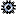line_index - Index Function

Syntax

... line_index( table_exp  ) ...

The table function line_index returns the number of the row found using the table expression table_exp with respect to the table index used. The return value has the type i.

The row itab_line found using the table expression must be defined by specifying a key. An index cannot be specified. Alongside single table expressions, table_exp can also handle chainings, whose result is a row of an internal table (i.e. they are closed using angle brackets). The result always refers to the row specified in the final angle bracket and the key read restrictions apply only to this angle bracket.

Within line_index, an explicitly specified table key in the table row table_line of the table expression is handled in the same way as a free search key specified for this table key.

The table index used makes reference to the specified key as follows:

• If a search key is specified without a table key being specified explicitly, the number of a found row is returned with respect to the primary table index; if a hashed table is specified, the value -1 is returned.
• If a table key is specified explicitly after KEY, the number of a found row is returned with respect to the assigned table index. If the key is a hash key, the value -1 is returned.

If the row in question is not found, no exception is raised. The value 0 is returned instead. In the case of chainings, of table expressions, the first unsuccessful from the left produces this value.

Notes

• An index specified in itab_line of the table expression is not possible here, since it does not make sense.
• The table expression is only used to check the existence of the row number specified row. No temporary result is created.
• The table function line_index can be considered as a short form of the statement READ TABLE with the addition TRANSPORTING NO FIELDS following by sy-tabix being evaluated. If a row is not found, 0 is always returned. sy-tabix, on the other hand, has the value where the row would be found after a binary search using READ TABLE.
• If a search key specified in table_line in the table expression covers the initial part of a secondary table key without being specified explicitly after KEY, a syntax check warning is produced (which can be hidden by a pragma), since the function is generally quicker if the secondary key is specified explicitly.
• As in other use cases for table expressions, line_index must be used carefully and no duplicate selections made. Generally speaking, it is best not to first check the row number using line_index and then read the row immediately using the index.
• The predicate function line_exists can be used to check the existence of a row. The table function line_index can also be used to check whether a row exists. If the row is found, the result for every table category is not equal to 0. A check performed on a value greater than 0, on the other hand, is only enough in the case of index tables or when sorted keys are used.

Example

The index function can be used on chain expressions as follows, where the nested internal table accessed is taken from the example for Chainings with Table Expressions. The first read returns the value 1 for the first row of the innermost table. The second read returns the value 0, since there is no row available for the index read on the outermost table.

DATA(idx1) = line_index(
itab[ 2 ][ 2 ][ KEY primary_key table_line = 7 ] ).

DATA(idx2) = line_index(
itab[ 9 ][ 2 ][ KEY primary_key table_line = 7 ] ).

Executable Example

ContinueInternal Tables, Index Function